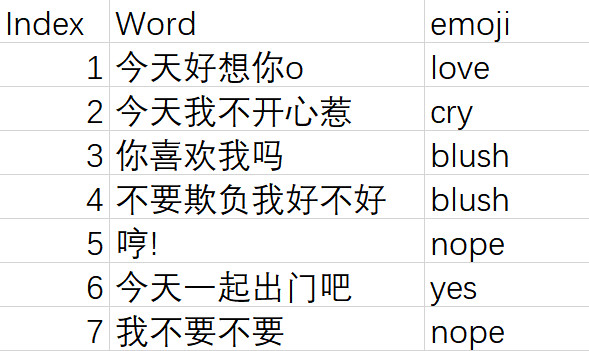（文末有彩蛋哦）## 第二步：生成Poisson分布随机数

### 方法 3 Data analysis toolVBA 里面实现是这样的，其中A, B, C, D, E, F为参数

• A = how many variables that are to be randomly generated
• B = number of random numbers generated per variable
• C = number corresponding to a distribution
• 1= Uniform
• 2= Normal
• 3= Bernoulli
• 4= Binomial
• 5= Poisson
• 6= Patterned
• 7= Discrete
• D = random number seed
• E = parameter of distribution (mu, lambda, etc.) depends on choice for C
1. = additional parameter of distribution (sigma, etc.) depends on choice for C

### 第三步：通过随机设定offset的值来实现随机生成对话的效果